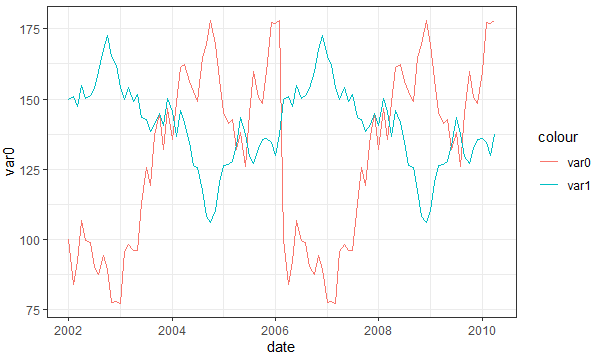2 views

A very newbish question, but say I have data like this:

test_data <-

data.frame(

var0 = 100 + c(0, cumsum(runif(49, -20, 20))),

var1 = 150 + c(0, cumsum(runif(49, -10, 10))),

date = seq(as.Date("2002-01-01"), by="1 month", length.out=100)

)

How can I plot both time series var0 and var1 on the same graph, with the date on the x-axis, using ggplot2? Bonus points if you make var0 and var1 different colors, and can include a legend!

I'm sure this is very simple, but I can't find any examples out there.

by

If the variables that you want to plot are few in number, then you can use geom_line() function with ggplot as follows:

ggplot(test_data, aes(date)) +

geom_line(aes(y = var0, colour = "var0")) +

geom_line(aes(y = var1, colour = "var1"))+

theme_bw()

Output: